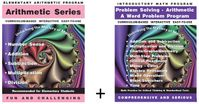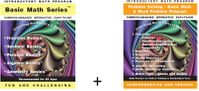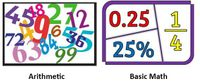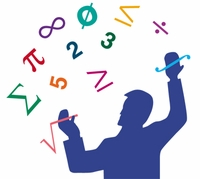Tutorial math and reading software for elementary and secondary arithmetic, basic math, algebra, geometry, precalculus plus GED, ABE, and CLEP preparation for elementary school, high school, college, adult education, and homeschool students.

# What is the difference between arithmetic and basic math?

## Product Description

What is the difference between arithmetic and basic math?

Arithmetic is a branch of mathematics that focuses on understanding numbers and performing mechanical manipulation of numbers using addition, subtraction, multiplication, division and problem solving using these skills. Arithmetic is the foundation of mathematics and is taught as a core subject in early education.

Basic math encompasses a range of fundamental mathematical concepts and skills, including arithmetic using the manipulation of arithmetic mechanics in fractions, decimals, percents, basic algebra and basic geometry with a higher level of problem solving. Skills in basic math are essential for navigating life's financial responsibilities as well as achieving success in higher mathematics.Arithmetic SeriesPLUS "Word Problems"Regular price: \$189.00Limited Time Offer: \$94.50Purchase all 6 Basic Math Programs for a Bundle PriceBasic Math Series + Word Problems for Basic MathRegular price: \$189.00Limited Time Offer: \$94.50What is the difference between Arithmetic and Basic Math?What is the difference between Arithmetic and Mathematics?